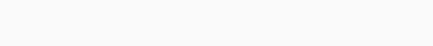#Aptitude Questions: Compound Interest Set 10

Hello Aspirants.

Welcome to Online Maths in AffairsCloud.com. Here we are creating question sample in Compound Interest, which is common for all the IBPS, SBI, RBI, IPPB, LIC and other competitive exams. We have included Some questions that are repeatedly asked in exams !!

Compound Interest

1. The compound interest on a certain sum of money at a certain rate per annum for two years is Rs. 4,100 and the simple interest on the same amount of money at the same rate for 3 years is Rs. 6000. Then the sum of money is :
1) Rs. 40,000
2) Rs. 36,000
3) Rs. 42,000
4) Rs. 50,000
5) none of these
Explanation :2. A sum of money was went at 10% per annum, compounded annually for 2 years. If the interest was compounded half yearly, he would have received Rs. 440.5 more. Find the sum.
1) Rs. 84000
2) Rs. 96000
3) Rs. 100000
4) Rs. 104000
5) Rs. 80000
Explanation :

[caption id="attachment_120878" align="alignnone" width="561"]Compound Interest Q2[/caption]

3. Ragnal, Rollo and Vik start a business by investing Rs 70,000 that earns them a profit of Rs 42,000 at the end of the year. Ragnar invests his share in the profit in a scheme that gives him 10% interest compounded annually and Rollo invests his share in a scheme that gives him 20% interest compounded annually. Ragnar gets Rs 2,520 as interest at the end of 2 years and Rollo gets an interest of Rs 4,200 at the end of one year. Find Vik’s investment in the business.
1) Rs 1,60,000
2) Rs 15,000
3) Rs 17,520
4) Cannot be determined
5) none of these
Explanation :4. Rs. 12200 was partly invested in Scheme A at 10% p.a. compound interest (compounded annually) for 2 years and partly in Scheme B at 10% p.a. simple interest for 4 years. Both the schemes give equal interests. How much was invested in Scheme A ?
1) Rs. 7500
2) Rs. 9000
3) Rs. 8000
4) Rs. 6050
5) Rs. 10000
Explanation :5. A man borrows Rs. 8000 at 20% compound rate of interest. At the end of each year he pays back Rs. 3000. How much amount should he pay at the end of the third year to clear all his dues?
1) Rs. 5492
2) Rs. 5552
3) Rs. 5904
4) Rs. 6933
5) None of these
Explanation :6. A money-lender borrows money at 4% per annum and lends it at 6% per annum compound interest compounded half yearly and thus gains Rs. 209 in a year. The amount of money he borrows, is:
1) Rs. 12,000
2) Rs. 11,500
3) Rs. 10,000
4) Rs. 9,500
5) none of these
Explanation :7. What sum will give Rs. 488 as the difference between simple interest and compound interest at 10% in 1 1/2 years compounded half yearly ?
1) Rs. 80,000
2) Rs. 72,000
3) Rs. 64,000
4) Rs. 68,000
5) None of these
Explanation :8. A certain sum is interested at compound. The interest accrued in the first two years is Rs. 544 and that in the first three years is Rs. 868. Find the rate per cent.
1) 12 1/2%
2) 7 1/2%
3) 17 1/2%
4) 25%
5) None of these
Explanation :9. A man gave 50% of his savings of Rs. 168200 to his wife and divided the remaining sum among his sons Abid and Bisth of 15 and 13 years of age respectively. He divided it in such away that each of his sons when they attain the age of 18 years, would receive the same amount of 5% compound interest per annum. The share of Bisth was:
1) Rs. 42050
2) Rs. 40000
3) Rs. 45000
4) Rs. 45500
5) None of these
Explanation :10. What sum of money at compound interest will amount to Rs. 2249.52 in 3 years, if the rate of interest is 3% for the first year, 4% for the second year and 5% for the third year ?
1) Rs. 20140
2) Rs. 1980
3) Rs. 2000
4) Rs. 2180
5) None of these